# Class 12 Maths NCERT Solutions for Chapter 9 Differential Equations Miscellaneous Exercise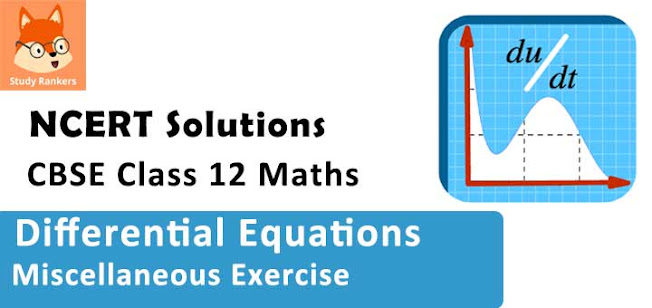### Differential Equations Miscellaneous Exercise Solutions

1. For differential equations given below, indicate its order and degree (if defined).
(i) (d2y/dx2 ) + 5x (dy/dx)2 – 6y = log x
(ii) (dy/dx)3  + 4 (dy/dx)2 + 7y = sin x
(iii) (d4y/dx4 ) - sin  (d3y/dx3 ) = 0

Solution

(i) The differential equation is given as :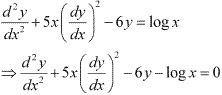The highest order derivative present in the differential equation is d2y/dx2. Thus, its order is two . The highest power raised to d2y/dx2 is one. Hence, its degree is one.

(ii) The differential equation is given as :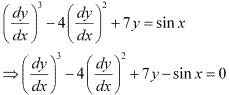The highest order derivative present in the differential equation is dy/dx. Thus, its order is one. The highest power raised to dy/dx is three. Hence, its degree is three.

(iii) The differential equation is given as :
(d4y/dx4) - sin(d3y/dx3) = 0
The highest order derivative present in the differential equation is d4y/dx4. Thus, its order is four.
However, the given differential equation is not a polynomial equation. Hence, its degree is not defined.

2. For given below, verify that the given function (implicit or explicit) is a solution of the corresponding differential equation.
(i) y = aex + be-x + x2 : x (d2y/dx2) + 2(dy/dx) - xy + x2 - 2 = 0
(ii) y = ex (a cos x + b sin x) : d2 y/dx2 - 2(dy/dx) + 2y = 0
(iii) y = x sin 3x : (d2y/dx2) + 9y - 6cos 3x = 0
(iv) x2 = 2y2 log y: (x2 + y2) dy/dx - xy = 0

Solution

(i) xy = aex + be-x + x2
Differentiating both sides w.r.t. x we get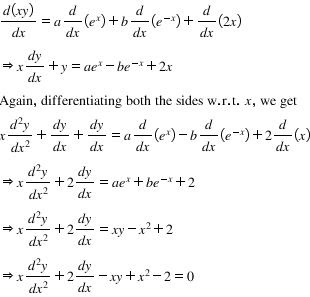Hence, the given function is a solution of the corresponding differential equation.

(ii) y = ex (a cos x + b sin x) = aex cos x + bex sin x.
Differentiating both sides with respect to x, we get :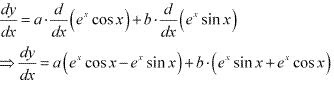⇒ dy/dx = (a + b)ex cos x + (b - a)ex sin x
Again, differentiating both sides with respect to x, we get ;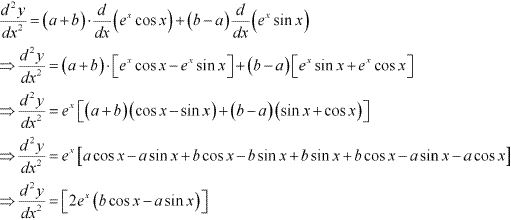Now, on substituting the values of d2y/dx2 and dy/dx in the L.H.S. of the given differential equation, we get :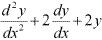= 2ex (b cos x - a sin x) - 2ex [(a + b) cos x + (b - a) sin x] + 2ex (a cos x + b sin x)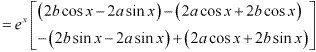= ex [(2b - 2a - 2b + 2a) cos x] + ex [(-2a - 2b + 2a + 2b) sin x]
= 0
Hence, the given function is a solution of the corresponding differential equation.

(iii) y = x sin3x
Differentiating both sides with respect to x, we get :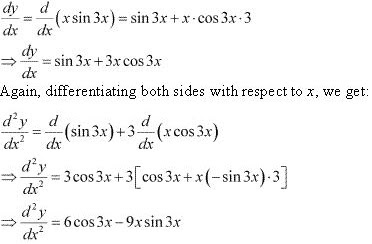Substituting the value of d2 y/dx2 in the L.H.S. of the given differential equation, we get :
d2y/dx2 + 9y - 6 cos 3x
= (6.cos 3x - 9x sin 3x) + 9x sin 3x - 6 cos 3x
= 0
Hence, the given function is a solution of the corresponding differential equation.

(iv) x2 = 2y2 log y
Differentiating both sides with respect to x, we get :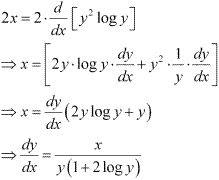Substituting the value of dy/dx in the L.H.S. of the given differential equation, we get :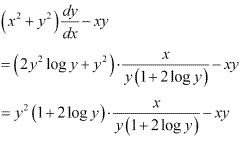= xy - xy
= 0
Hence, the given function is a solution of the corresponding differential equation.

3. Form the differential equation representing the family of curves given by (x – a)2 + 2y2 = a2, where a is an arbitrary constant.

Solution

(x - a)2 + 2y2 = a2
⇒ x2 + a2 - 2ax + 2y2 = a2
⇒ 2y2 = 2ax - x2 ...(1)
Differentiating with respect to x, we get :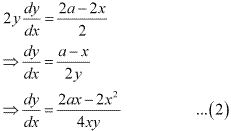From equation (1), we get :
2ax = 2y2 + x2
On substituting this value in equation (2), we get :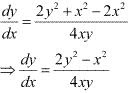Hence, the differential equation of the family of curves is given as dy/dx = (2y2 - x2)/4xy.

4. Form the differential equation of the family of circles in the first quadrant which touch the coordinate axes.

Solution

(x3 - 3xy2)dx = (y3 - 3x2 y)dy
⇒ dy/dx = (x3 - 3xy2)/(y3 - 3x2 y) ...(1)
This is a homogeneous equation. To simplify it, we need to make the substitution as :
y = vx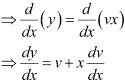Substituting the values of y and dy/dx in equation (1), we get :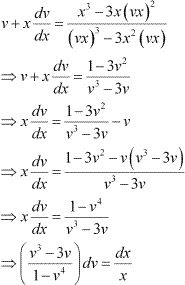Integrating both sides, we get :Substituting the values of I1 and I2­ in equation (3), we get :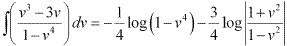Therefore, equation (2) becomes: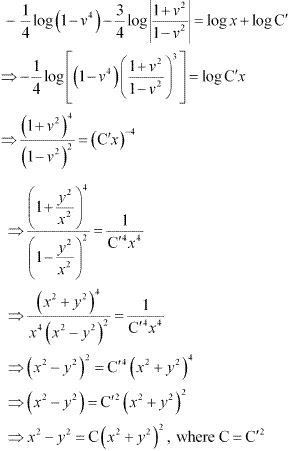Hence, the given result is proved.

5. Prove that x2 – y2 = c (x2 + y2)2 is the general solution of differential equation (x3 – 3xy2) dx = (y3 – 3x2y) dy, where c is a parameter.

Solution

The equation of a circle in the first quadrant with centre (a, a) and radius (a) which touches the coordinate axes is :
(x - a)2 + (y - a)2 = a2 ...(1)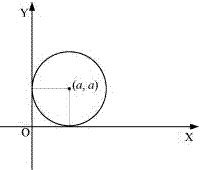Differentiating equation (1) with respect to x, we get :
2(x - a) + 2(y - a) dy/dx = 0
⇒(x - a)+ (y - a)y' = 0
⇒ x - a + yy' - ay' = 0
⇒ x + y y' - a(1 + y') = 0
⇒ a = (x + yy')/(1 + y')
Substituting the value of a in equation (1), we get :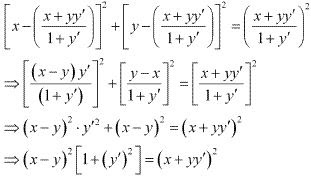Hence, the required differential equation of the family of circles is
(x - y)2 [1 +(y')2 ] = (x + yy')2.

6. Find the general solution of the differential equation dy/dx + √(1 - y2)/(1 - x2) = 0

Solution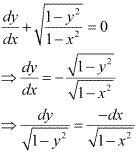Integrating both sides, we get :
sin-1y = -sin-1x + C
⇒ sin-1x + sin-1y =  C

7. Show that the general solution of the differential equation dy/dx + (y2 + y + 1)/(x2 + x + 1) = 0 is given by (x + y + 1) = A (1 - x - y - 2xy), where A is parameter.
Solution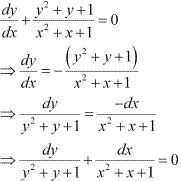Integrating both sides, we get :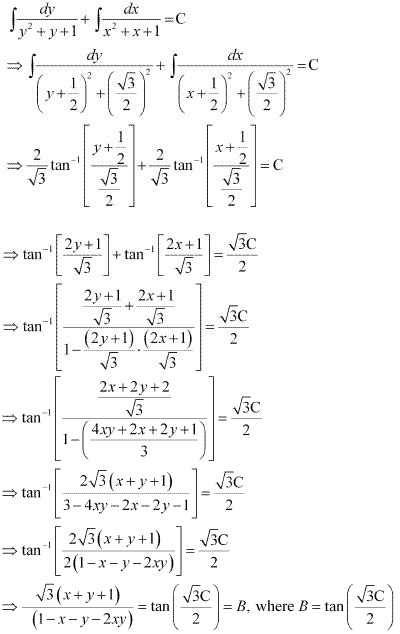⇒ x + y + 1 = B/√3(1 - xy - 2xy)
⇒ x + y + 1 = A(1 - x - y - 2xy), where A = B/√3
Hence, the given result is proved.

8. Find the equation of the curve passing through the point (0, π/4), whose differential equation is sin x cos y dx + cos x sin y dy = 0.
Solution
The differential equation of the given curve is :
sin x cos y dx + cos x sin ydy = 0
⇒ (sin x cos ydx + cos x sin ydy)/(cos x cos y) = 0
⇒ tan x dx + tan y dy = 0
Integrating both sides, we get :
log (sec x) + log (sec y) =log C
log (sec x .sec y) = log C
⇒ sec x .sec y = C ...(1)
The curve passes through point (0, π/4).
∴ 1 × √2 = C
⇒ C = √2
On substituting C = √2 in equation (1), we get :
sec x . sec y = √2
⇒ sec x . 1/cos y = √2
⇒ cos y = sec x/√2
Hence, the required equation of the curve is cos y = sec x/√2.

9. Find the particular solution of the differential equation (1 + e2x)dy + (1 + y2) ex dx = 0, given that y = 1 when x = 0.
Solution
(1 + e2x )dy + (1 +y2 )ex dx = 0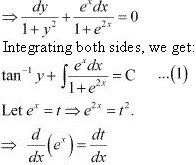⇒ ex = dt/dx
⇒ ex dx = dt
Substituting these values in equation (1), we get :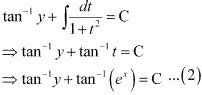Now, y = 1 at x = 0.
Therefore, equation (2) becomes:
tan-1 1 + tan-1 1 = C
⇒ π/4 + π/4 = C
⇒ C = π/4
Substituting C = π/4 in equation (2), we get :
tan-1 y + tan-1 (ex) = π/2
This is the required particular solution of the given differential equation.

10. Solve the differential equation yex/y dx = (xex/y + y2)dy (y ≠ 0)
Solution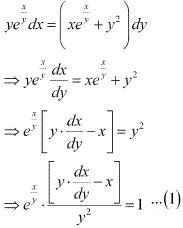Let ex/y = Z
Differentiating it with respect to y, we get :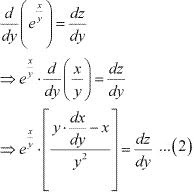From equation (1) and equation (2), we get :
dz/dy = 1
⇒ dz = dy
Integrating both sides, we get :
z = y + C
⇒ ex/y = y + C

11. Find a particular solution of the differential equation (x – y) (dx + dy) = dx – dy, given that y = –1, when x = 0. (Hint: put x – y = t)
Solution
(x - y)(dx + dy) = dx - dy
⇒ (x - y + 1)dy = (1 - x + y)dx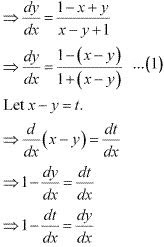Substituting the values of x - y and dy/dx in equation (1), we get :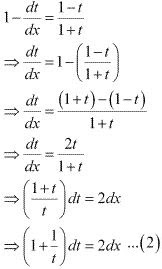Integrating both sides, we get :
t + log |t| = 2x + C
⇒ (x - y) + log |x - y| = 2x + C
⇒ log |x - y| = x + y + C ...(3)
Now, y = -1 at x = 0 . Therefore, equation (3) becomes :
log 1 = 0 - 1 + C
C = 1
Substituting C = 1 in equation (3) we get :
log |x - y| = x + y + 1
This is the required particular solution of the given differential equation.

12. Solve the differential equation [(e-2√x /√x) – y/√x] dx/dy = 1(x ≠ 0)
Solution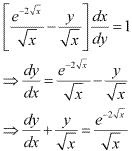This equation is a linear differential equation of the form
dy/dx + Py = Q, where p = 1/√x and Q = e-2√x /√x .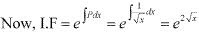The general solution of the differential equation is given by,
y(I.F.) = ∫(Q× I.F.)dx + C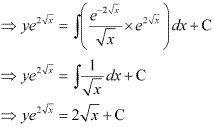13. Find a particular solution of the differential equation dy/dx + y cot x = 4x cosec x (x ≠ 0), given that y = 0 when x = π/2
Solution
The given differential equation is :
dy/dx + y cot x = 4 x cosec x
This equation is a linear differential equation of the form
dy/dx + py = Q, where p = cot x and Q = 4x cosec x.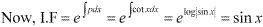The general solution of the given differential equation is given by,
y(I.F.) = ∫(Q × I.F.)dx + C
⇒ y sin x = ∫(4x cosec x . sin x)dx + C
⇒ y sin x = 4 ∫x dx + C
⇒ y sin x = 4. x2 /2 + C
⇒ y sin x = 2x2 + C  ...(1)
Now, y = 0 at x = π/2
Therefore, equation (1) becomes :
0 = 2 × (π2/4) +  C
⇒ C = -(π2/2)
Substituting C = -(π2/2) in equation (1), we get :
y sin x = 2x2 - (π2/2)
This is the required particular solution of the given differential equation.

14. Find a particular solution of the differential equation (x + 1) dy/dx = 2e-y - 1, given that y = 0 when x = 0
Solution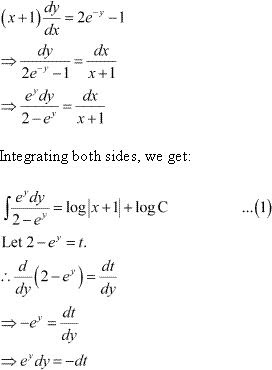Substituting this value in equation (1), we get :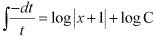⇒ - log |t| = log |C(x + 1)|
⇒ - log |2 - ey| = log |C(x + 1)|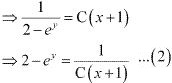Now, at x = 0 and y = 0, equation (2) becomes :
⇒ 2 - 1 = 1/C
⇒ C = 1
Substituting C = 1 in equation (2), we get :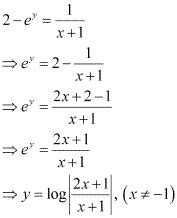This is the required particular solution of the given differential equation.

15. The population of a village increases continuously at the rate proportional to the number of its inhabitants present at any time. If the population of the village was 20000 in 1999 and 25000 in the year 2004, what will be the population of the village in 2009?
Solution
Let the population at any instant (t) be y.
It is given that the rate of increase of population is proportional to the number of inhabitants at any instant .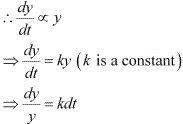Integrating both sides, we get :
log y + kt + C  ...(1)
In the year 1999, t = 0 and y = 20000.
Therefore, we get :
log 20000 = C ...(2)
In the year 2004, t = 5 and y = 25000.
Therefore, we get :
Log 25000 = k. 5 + C
⇒ log 25000 = 5k + log 20000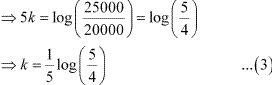In the year 2009, t = 10 years.
Now, on substituting the values of t, k, and C in equation (1), we get :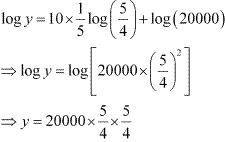⇒ y = 20000× 5/4 × 5/4
⇒ y = 31250
Hence, the population of the village in 2009 will be 31250.

16. The general solution of the differential equation (ydx - xdy)/y = 0
(A) xy = C
(B) = Cy2
(C) = Cx
(D) y = Cx2
Solution
The given differential equation is :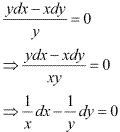Integrating both sides, we get :
log |x| - log |y| = log k
⇒ log |x/y| = log k
⇒ x/y = k
⇒ y = (1/k)x
⇒ y = Cx where C = 1/x
Hence, the correct answer is C.

17. The general solution of a differential equation of the type dx/dy + P1x = Q is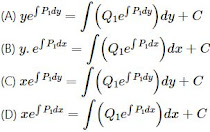Solution
The integrating factor of the give differential equation dx/dy + P1x = Q1 is e∫p1dy
The general solution of the differential equation is given by,
x(I.F.) = ∫(Q × I.F.) dy + C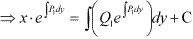Hence, the correct answer is C.

18. The general solution of the differential equation exdy + (y ex + 2x) dx = 0 is
(A xey + x2 = C
(B) xey + y2 = C
(C) yex + x2 = C
(D) ye+ x2 = C
Solution
The given differential equation is :
ex dy + (yex + 2x)dx = 0
⇒ ex (dy/dx) + yex + 2x = 0
⇒ dy/dx + y = -2xe-x
This is a linear differential equation of the form
dy/dx + Py = Q, where P = 1 and Q = -2xe-x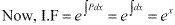The general solution of the given differential equation is given by,
y(I.F.) = ∫(Q × I.F.) dx + C
⇒ yex  = ∫(-2xe-x .ex) dx + C
⇒ yex = -∫2xdx + C
⇒ yex = -x2 + C
⇒ yex + x2 = C
Hence, the correct answer is C.